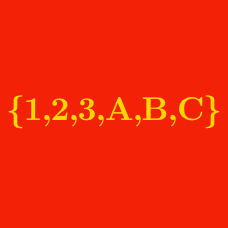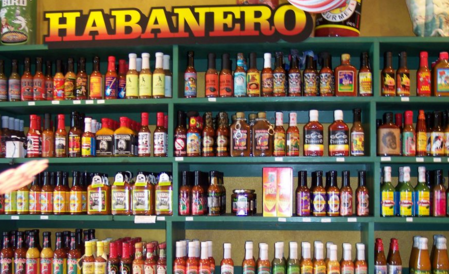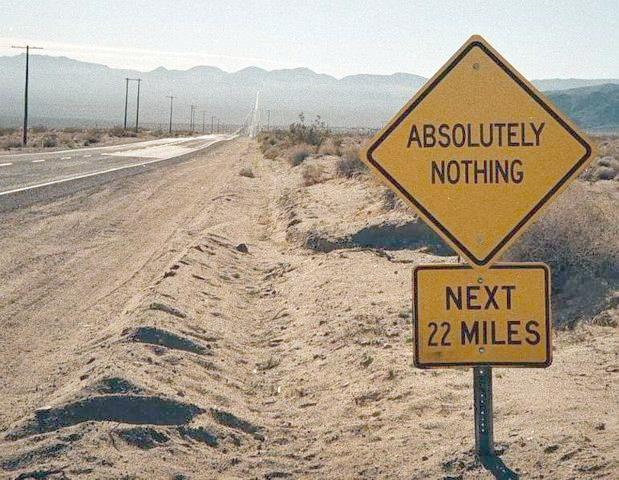Probability

# Set Notation: Level 2 Challenges

How many sets A exist for which $\{1,2\} \subseteq A \subseteq \{1,2,3,4,5\}$ ?Solve for $X$.

As a true connoisseur of capsaicin, you can taste the presence of every single one your $X$ chili species distinctly in any chili sauce. So there are a LOT of chili sauces in your kitchen. In fact, your kitchen is basically filled with the $8192$ tiny, semi-explosive chili sauce bottles you own. Why so many? -- because you have every kind of chili sauce that uses any combination of any number of your chilies (including one INSANE chili sauce that has all $X$ of the kinds of pepper you love in it!!!! -- whenever you use it, there's a 20% chance of spontaneous combustion of the food it's put on.) You also have exactly one chililess chili sauce among the $8192$, just to complete the set... but anyone who uses it is a dodo bird.Calculate |$\mathcal{P}(\mathcal{P}(A))$| if A is the Empty Set and $\mathcal{P}(X)$ means "the power set of set $X$."

In how many subsets of $\{1,2,3,4,5\}$ is the sum of the largest element and the smallest element equal to 6?

X is a subset of Y and Y is a subset of Z. Which of the following statements must be true?

(A)$\ \$ If 1 is in Y, then 1 is in X.
(B)$\ \$ If 2 is in Z, then 2 is in X.
(C)$\ \$ If 3 is in Z, then 3 is in Y.
(D)$\ \$ If 4 is in X, then 4 is in Z.
(E)$\ \$ None of the above

×